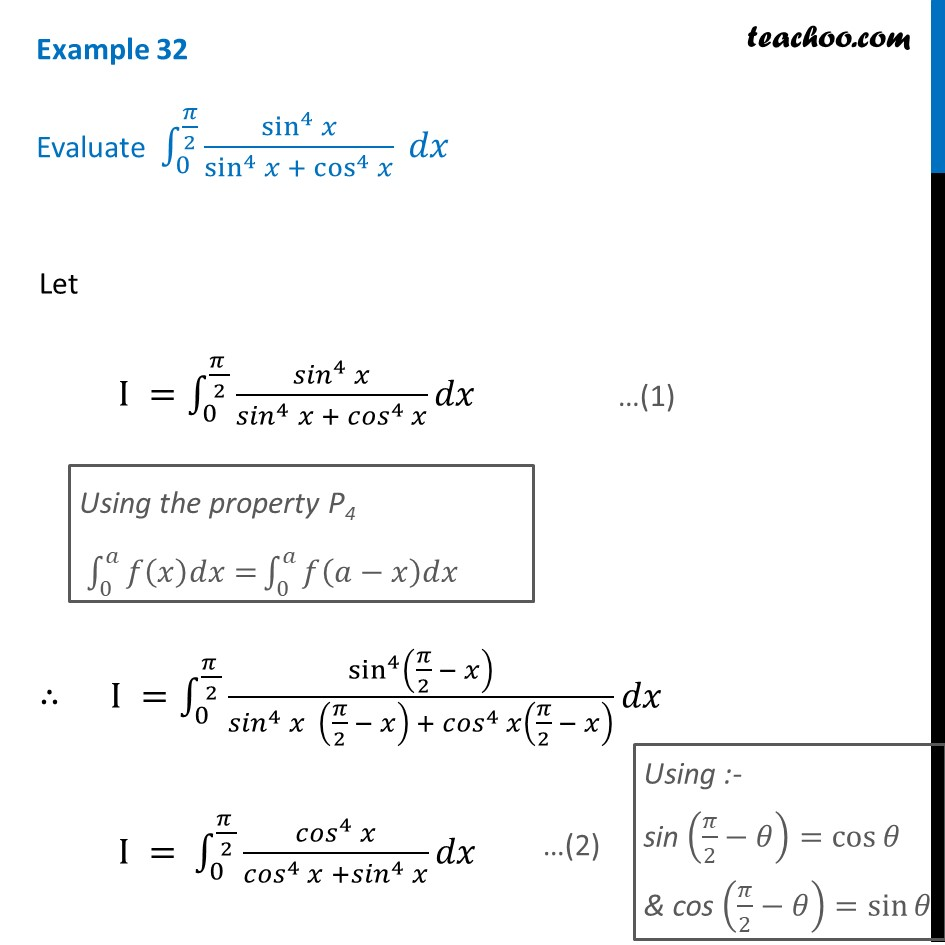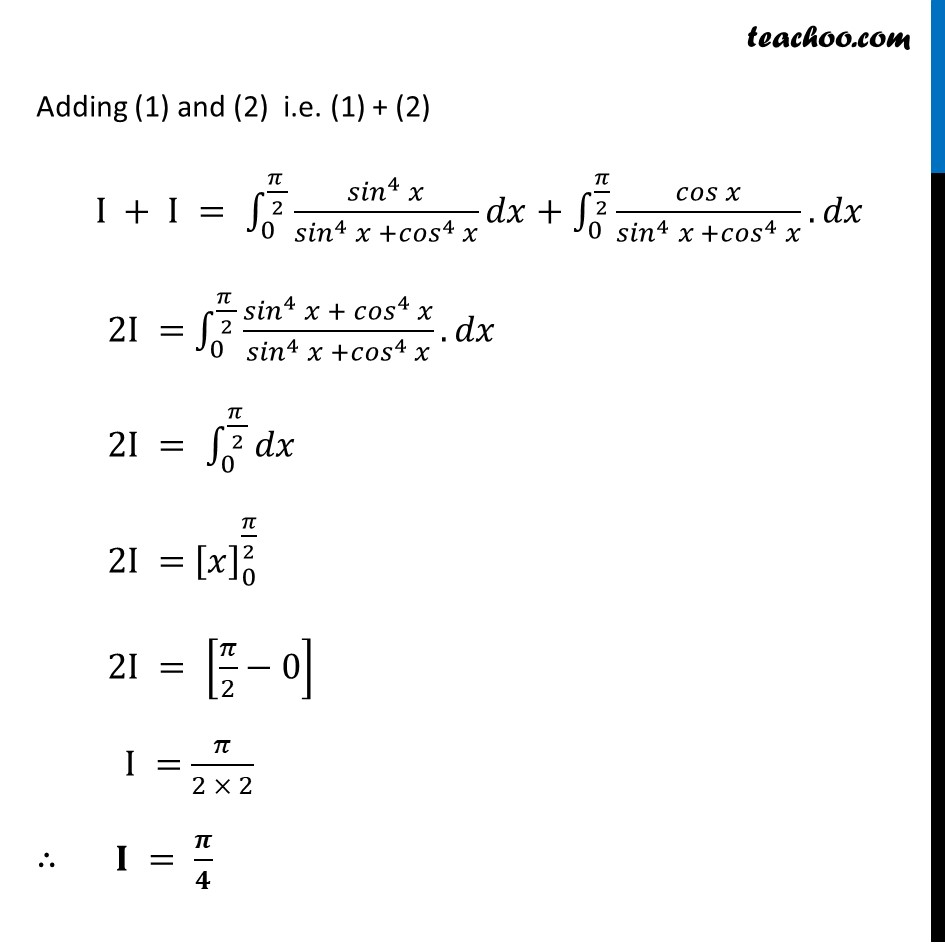Definite Integration by properties - P4

Chapter 7 Class 12 Integrals
Concept wiseLearn in your speed, with individual attention - Teachoo Maths 1-on-1 Class

### Transcript

Example 32 Evaluate ∫_0^(𝜋/2)▒sin^4⁡𝑥/(sin^4⁡𝑥 + cos^4⁡𝑥 ) 𝑑𝑥 Let I =∫_0^((𝜋 )/2)▒〖(〖𝑠𝑖𝑛〗^4 𝑥)/〖〖𝑠𝑖𝑛〗^4 𝑥〗⁡〖+ 〖𝑐𝑜𝑠〗^4 𝑥〗 𝑑𝑥〗 ∴ I =∫_0^((𝜋 )/2)▒sin^4⁡(𝜋/2 − 𝑥)/(〖〖𝑠𝑖𝑛〗^4 𝑥〗⁡〖 (𝜋/2 − 𝑥) 〗+ 〖〖𝑐𝑜𝑠〗^4 𝑥〗⁡(𝜋/2 − 𝑥) ) 𝑑𝑥 I = ∫_0^((𝜋 )/2)▒(〖𝑐𝑜𝑠〗^4 𝑥)/〖〖𝑐𝑜𝑠〗^4 𝑥〗⁡〖+〖𝑠𝑖𝑛〗^4 𝑥〗 𝑑𝑥 Adding (1) and (2) i.e. (1) + (2) I + I = ∫_0^((𝜋 )/2)▒(〖𝑠𝑖𝑛〗^4 𝑥)/(〖𝑠𝑖𝑛〗^4 𝑥 +〖𝑐𝑜𝑠〗^4 𝑥) 𝑑𝑥+∫_0^(𝜋/2)▒〖(𝑐𝑜𝑠 𝑥)/(〖𝑠𝑖𝑛〗^4 𝑥 +〖𝑐𝑜𝑠〗^4 𝑥).〗 𝑑𝑥 2I = ∫_0^((𝜋 )/2)▒〖(〖𝑠𝑖𝑛〗^4 𝑥 + 〖𝑐𝑜𝑠〗^4 𝑥)/(〖𝑠𝑖𝑛〗^4 𝑥 +〖𝑐𝑜𝑠〗^4 𝑥).〗 𝑑𝑥 2I = ∫_0^((𝜋 )/2)▒𝑑𝑥" " 2I = [𝑥]_0^(𝜋/2) 2I = [𝜋/2−0] I = 𝜋/(2 × 2) ∴ 𝐈 = 𝝅/𝟒